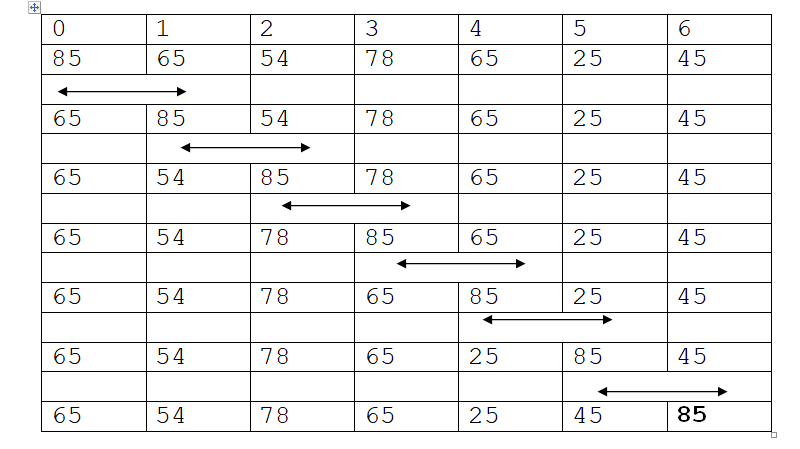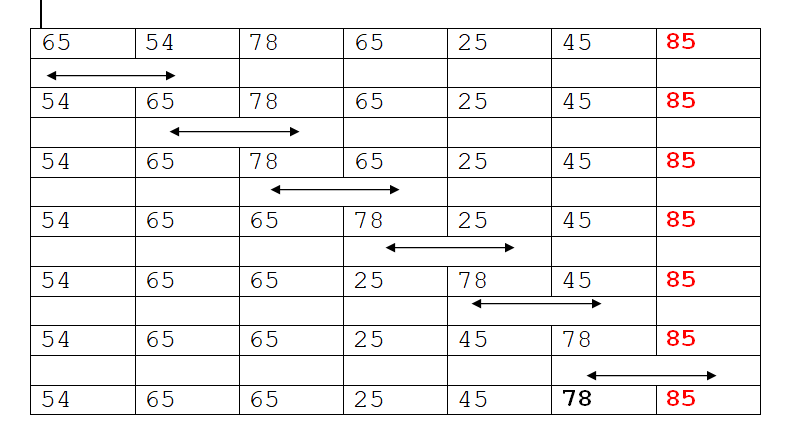# Bubble Sort in C, C++, Python, Java, Php

## Sorting

Sorting is the operation of arranging the records of a table/list/array in a particular order, either in ascending or descending order.

## Bubble sort

The basic idea in bubble sort is to compare two successor elements and interchange them if they are not in proper order. After each pass the largest unsorted element is placed at its correct position.

If there are “n” elements then
After the first pass the largest element is placed at (n-1) th position. After the second pass the 2nd largest element is placed at n-2 th position and so on.
All the elements will be sorted after a maximum of n-1 passes.

But it is possible that the elements may get sorted before n-1 passes. So there should be a way to detect that the array is sorted or not. And for the same we use a variable swapped.

### C Implementation Of Bubble Sort    , C++ Implementation Of Bubble Sort    , Python Implementation Of Bubble Sort    , Java Implementation Of Bubble Sort    , PHP Implementation Of Bubble Sort

Example:
Elements : 85,65,54,78,65,25,45
Pass 1:Pass 2:and so on

## Complexity of bubble sort

### Best Case:

In the best case when the elements are already sorted then there will be only one comparison per pass so there will be total of n comparisons. I.e. order of n i.e. O (n).

### Worst case:

In this case the array list will be an invert list (i.e. 5,4,3,2,1,0) . To move from first element to the end of the array , n-1 times the swapping procedures is to be called. Every other element in the list will also move one location towards the start or end of the loop on every iteration. Thus “n” times the outer loop will iterate and n(n-1) times the inner loop will iterate to sort an inverted array.
F(n0=(n(n-1))/2=O(n2) (order of n square)

### Average Case:

Average case is very difficult to analyze than the other cases. In this case the data are randomly placed in the list. The exact time complexity can be calculated only if we know the number of iterations, comparisons and swapping. In general , the complexity of average case is :
f(n)=(n(n-1))/2=O(n2). (order of n square)

It is easy to learn.
Few lines of code
It works very well on already sorted lists or list with just a few permutations.

It is not effective for large numbers of sorting elements.
Complexity is O(n2) (order of n square)

C Bubble Sort

### C Implementation of Bubble Sort

```/* bubble sort*/
#include<stdio.h>
#include<conio.h>
void bubble( int a[], int n);
void main()
{
int a,i,n;
clrscr();
printf("enter the total number of elements "");
scanf("%d",&n);
for(i=0;i>a[i];
}
bubble(a,n);
printf("\nsorted elements list is \n");
for(i=0;i<n;i++)
{
printf("%d\n",a[i]);
}
getch();
}
void bubble(int a[],int n)
{
int i,t,j;
for(i=0;ia[j+1])
{
t=a[j];
a[j]=a[j+1];
a[j+1]=t;
}
}
}
}```

C++ Bubble Sort

### C++ Implementation of Bubble Sort

```/* bubble sort*/
#include<iostream.h>
#include<conio.h>
void bubble( int a[], int n);
void main()
{
int a,i,n;
clrscr();
cout<<"enter the total number of elements "; cin>>n;
for(i=0;i>a[i];
}
bubble(a,n);
cout<<"\nsorted elements list is \n";
for(i=0;i<n;i++)
{
cout<<a[i]<<endl;
}
getch();
}
void bubble(int a[],int n)
{
int i,t,j;
for(i=0;ia[j+1])
{
t=a[j];
a[j]=a[j+1];
a[j+1]=t;
}
}
}
}```

Python Bubble Sort

### Python Implementation of Bubble Sort

```#(bubble_sort.py)
def bubble_sort(n1):
for i in range(len(n1) - 1, 0, -1):
for j in range(0, i):
if(n1[j ] > n1[j+1]):
n1[j], n1[j + 1] = n1[j + 1], n1[j]
n = input('Enter the list of numbers: ')
n1=n.split()
convert all numbers to int
n1 = [int(x) for x in n1]
bubble_sort(n1)
print('Sorted list: ', end='')
print(n1)```

Output:

Enter the list of numbers: 2 5 9 3 47 5 6
Sorted list: [2, 3, 5, 5, 6, 9, 47]
>>>

### C Implementation Of Bubble Sort    , C++ Implementation Of Bubble Sort    , Python Implementation Of Bubble Sort    , Java Implementation Of Bubble Sort    , PHP Implementation Of Bubble Sort

Important PagesNumber Of Visitors